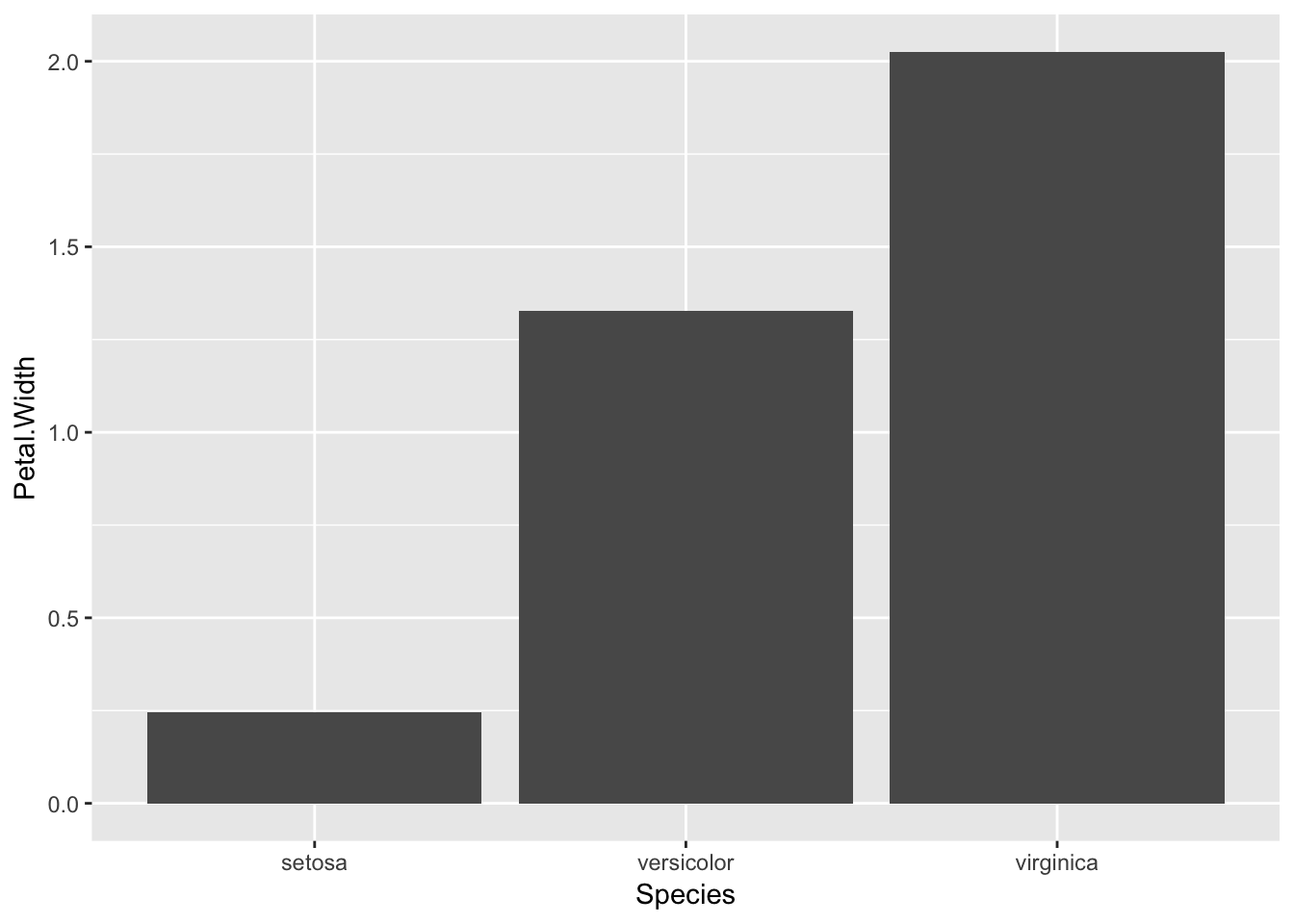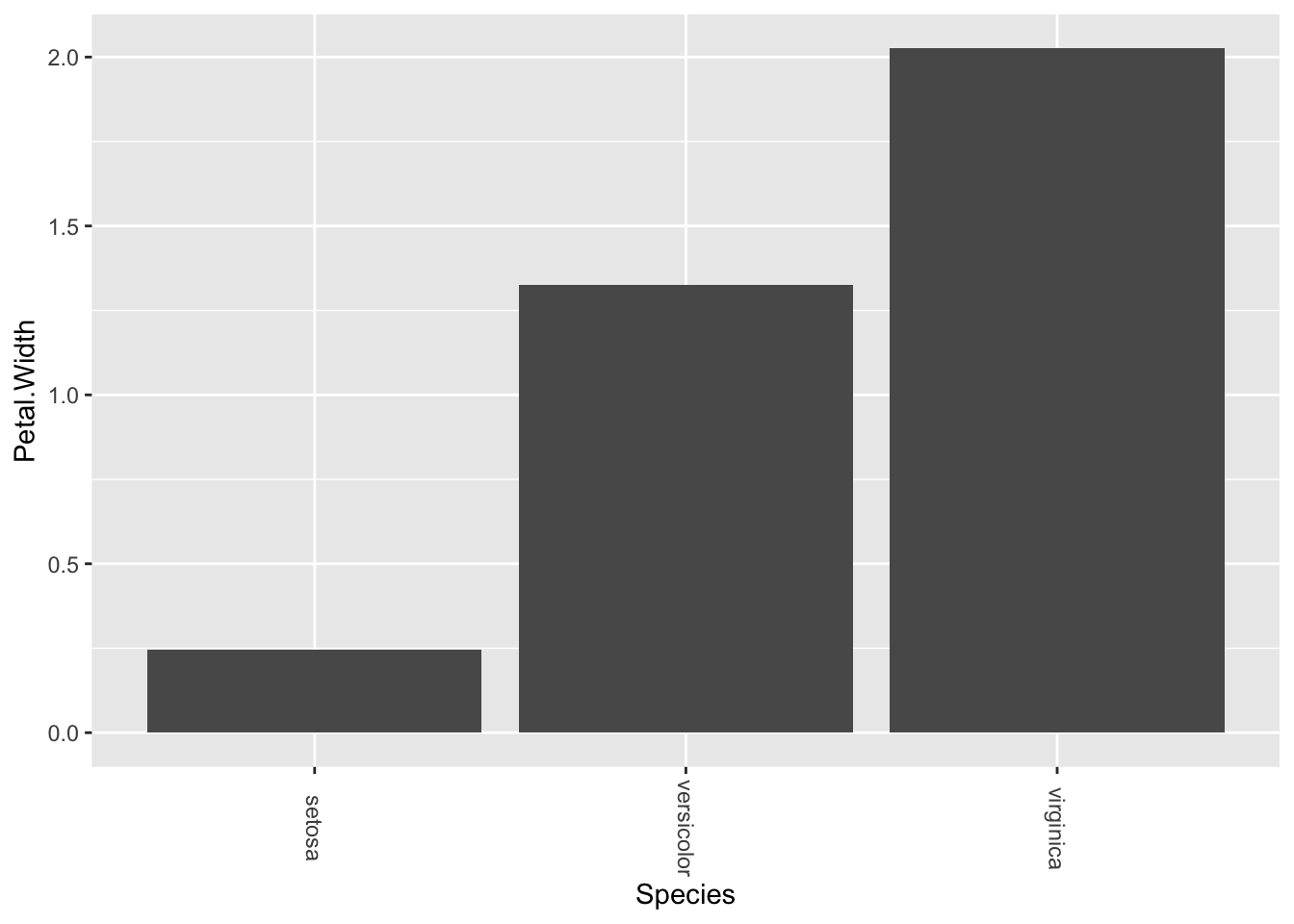# 1 Goal

The goal of this tutorial is to rotate the x axis text.

# 2 Data import

# First we load the libraries
library(ggplot2)
library(dplyr)

# We are going to use the iris dataset
data("iris")

# 3 Creating the histogram

# We want to plot the average petal width per species
iris_avg_pw <- iris %>% group_by(Species) %>% summarise(Petal.Width = mean(Petal.Width)) %>% as.data.frame()

# Let's plot
ggplot(data = iris_avg_pw) + geom_col(aes( x = Species, y = Petal.Width))# 4 Rotating the x axis’ text

# Now we can rotate the axis' texts
ggplot(data = iris_avg_pw) + geom_col(aes( x = Species, y = Petal.Width)) +
theme(axis.text.x=element_text(angle =- 90, vjust = 0.5))# vjust = 0.5 puts the rotated text in the center of the tick

# 5 Conclusion

In this tutorial we have learnt how to rotate the x axis’ text. This could be useful for example when we want to plot dates and the text in the axis overlap.